Function Repository Resource:

SmoothIntegerQ

Test whether the prime factors of an integer are less than a given threshold

Contributed by: Kevin Reiss and Zachary Shelton
 ResourceFunction["SmoothIntegerQ"][n,p] returns True if there is no prime factor of n greater than p and False otherwise. ResourceFunction["SmoothIntegerQ"][p] represents an operator form of ResourceFunction["SmoothIntegerQ"] that can be applied to an integer.

Details

An integer is said to be p-smooth if its largest prime factor is less than or equal to p.
p can be any positive real; the largest prime less than or equal to p is used.
For negative integers n, n is considered p-smooth if Abs[n] is p-smooth.

Examples

Basic Examples (2)

Show that 54 is 7-smooth:

 In:=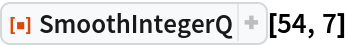Out=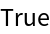54 is not 2-smooth, since its prime factors are 2 and 3:

 In:=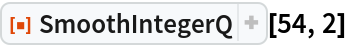Out=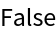Scope (2)

The following is an operator which tests whether an integer is 7-smooth:

 In:=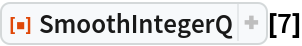Out=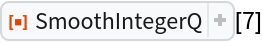In:=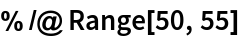Out=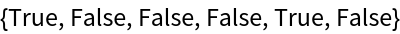An integer is p-smooth for non-prime p if it is p-smooth for p being the greatest prime less than p:

 In:=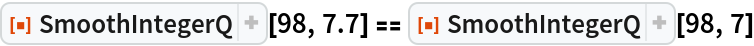Out=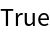π - smooth numbers are the same as 3-smooth numbers:

 In:=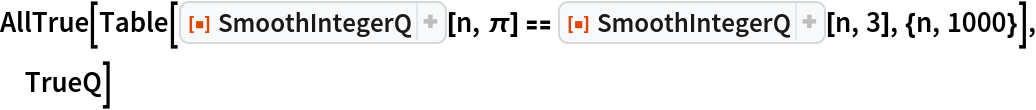Out=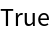Applications (1)

Print the 7-smooth numbers less than or equal to 100:

 In:=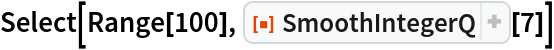Out=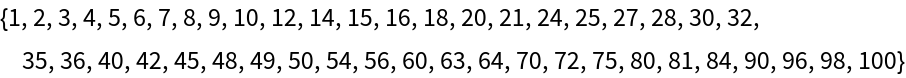Properties and Relations (3)

A negative integer is considered smooth if its absolute value is smooth:

 In:=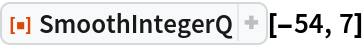Out=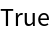Test that if n is 5-smooth, then so is -n for n up to 100:

 In:=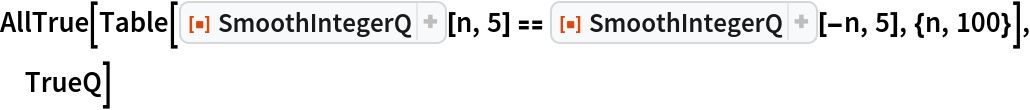Out=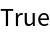Any input besides an integer in the first argument gives False:

 In:=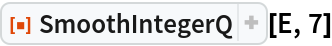Out=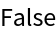In:=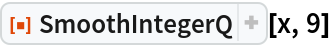Out=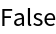Negative values for the second input always return False:

 In:=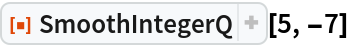Out=In:=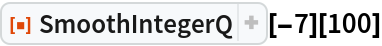Out=Neat Examples (2)

Calculate the number of "humble numbers" (7-smooth integers) with 1, 2, 3, 4 and 5 digits:

 In:=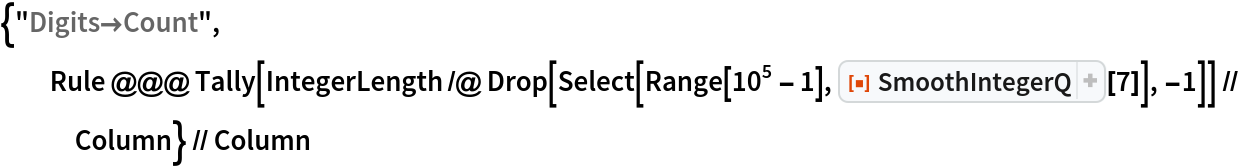Out=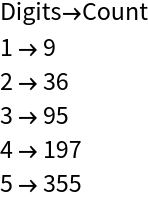123200 and 123201 comprise the largest consecutive pair that are both 13-smooth:

 In:=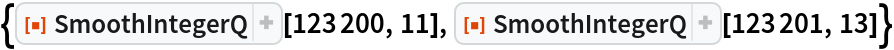Out=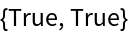Author Notes

This Function Repository submission was created live as a part of the Kevin + Zach livecoding series: Building a function the #WolframWay–YouTube.